# Modified Biogeography Based Optimization

2828 words (11 pages) Essay

29th Mar 2018 Computer Science Reference this

Tags:

Disclaimer: This work has been submitted by a university student. This is not an example of the work produced by our Essay Writing Service. You can view samples of our professional work here.

Any opinions, findings, conclusions or recommendations expressed in this material are those of the authors and do not necessarily reflect the views of UKEssays.com.

Modified Biogeography Based Optimization and enhanced simulated annealing on Travelling Tournament problem.

Abstract: This paper shows the implementation of Modified BBO and Extended BBO on Travelling Tournament Problem. We modified the migration step of BBO by using probabilistic measures into it. Conventional BBO is used to solve deterministic problems but when we are dealing with real world problems which are non deterministic Conventional BBO failed to achieve the desired/expected results. Modified BBO is able to handle non deterministic problems which occurs in TTP and considered it as noise. The physical significance of noise in our modified solution is any existing parameter which can affect the fitness of the habitat. We also implemented various models of Extended BBO (Linear and Non linear models) on TTP to achieve desirable results. In this paper we compare the performance of our modified BBO to conventional BBO on TTP problem and compare results with previous methodologies.

1. Introduction

BBO is Global optimization method which represents organism distribution in our biological system in terms of mathematical model .BBO is an evolutionary algorithm whose working principle is based upon migration mechanisms of species from one habitat to other depending upon the fitness of the habitat which are favorable to them .The habitat which have high HSI (high suitability index ) have high value of species count .Therefore habitat which have high value of HSI have high emigrating rate; it is ready to send its SIV to other habitat, while the habitat having low value of HSI have low value of species count and their immigrating rate is high ;that is it as ready to accept species towards itself .HSI of a habitat can be affected on the basis of SIV (suitability index variables) which are independent variables.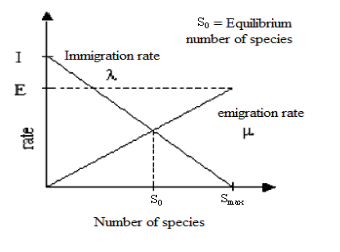The above diagram illustrates the basic mechanism and relation between immigration curve and emigration curve. Here I is maximum Immigration rate, E is maximum emigration rate,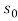is equilibrium number of species,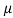is emigration rate and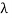is Immigration rate.

Modified BBO for TTP:

Firstly the question arises why there is need of Modification of BBO .We are dealing with biological issues which are dynamic in nature so we have to modified our solution which can take care all dynamic constraints of nature.

Let we have two habitats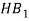and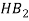.These habitats have their fitness’s as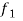and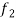.Let noise involved in two habitats are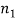and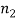.Due to affect of noise the measured fitness is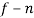instead of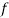.If we considerhas more fitness than,and let n1 has huge value than n2 and both high value than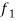and.Therefore the overall fitness becomes: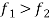1.1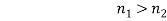1.2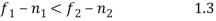Therefore HB1 accepts the SIV from HB2 as condition of BBO gets satisfied as immigrating habitat fitness is less than emigrating habitat. But population of HB1 is already high due to its high HSI because its fitness is more if don’t consider noise .this immigration should not be done .The BBO migration procedure will corrupted .That’s why we need to modify it.

In order to calculate the uncertainties, we use the concept of noisy BBO[].

U=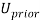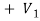1.4

E=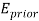+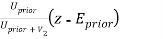1.5

U =1.6

Where U is the uncertainty of the state estimate, m is the estimated fitness, z is the measured fitness,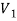is the variance of the process noise, and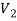is the variance of the observation noise. The uncertainty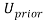and the estimated fitnessare the values from the previous iteration step before the most recent fitness measurement is updated. The process noise is assumed to be zero, therefore the uncertainty U is only relatedand.

U =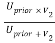1.7

U =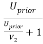1.8

Because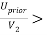0, now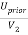+1 > 1. Therefore+1<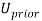.With each step in the Kalman algorithm, the uncertainty U will be reduced according toand. Small value of uncertainty leads to high accuracy of estimated fitness.

If limit tends to infinity, than Kalman filter gives an estimate value of the fitness which is equal to the real value.

Proposed Modified BBO algorithm:

1. Select habitat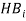with the Probability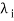.
2. Ifis selected
3. For j=1 to n
4. Select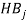with the probability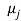.
5. Ifis selected
6. Use rand (0, 1) to select SIV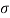from the habitat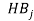and pass it through Modification phases.
7. Choose the best feasible solution based on optimal selection from the output of three Modification Phases.
8. Replace selected SIV with9. End of if
10. End
11. End of if

The above Algorithm solves all the issues that is related with Deterministic Problems. We Map this approach to different variants of BBO that can be classified as its Models.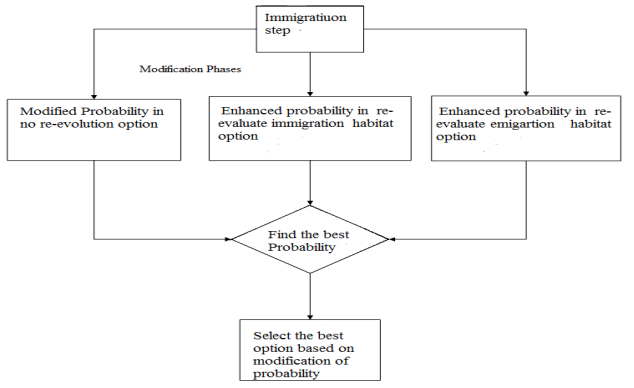Equations used :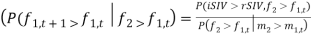The above equation is generalization of Baye’s rule.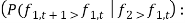Probability of a habitat with fitness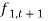after accepting a selected SIV greater than fitness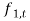given that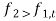.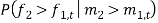is simply equal to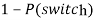where P(switch) is given by :

When x > y we obtain: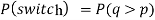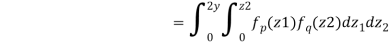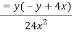if x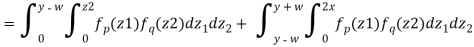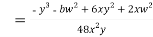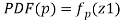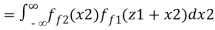The PDF of p is as follows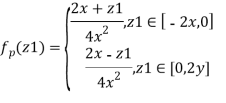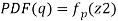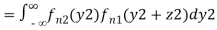The PDF of q is as follows.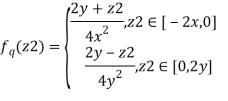In the Modification step we talked about three ways by which we can increase the performance of BBO. These three ways can be described as:1. No-reevaluation phase: In this phase we have two Habitats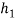as immigrating habitat and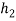act as emigrating habitat. We consider two instances ofas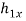and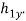Firstly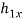is going to accept optimal SIV fromand then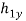accept another best suitable SIV from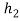and after that their performance get measured on the probabilistic measures as: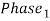=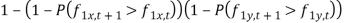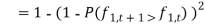1. Immigrating Habitat Re-evaluation: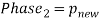(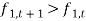)

1. Emigrating habitat Re-evaluation: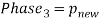()

From the above phases we choose the best option for the immigration step.

Secondly we Map this Modification approach to all the variants of Extended BBO and implement it on TTP problem. We Modified the Immigration step and apply this Modification to all the linear and non linear Models of BBO to check whether we are able to achieve the optimal results or not. We Test our algorithm to obtain various results which provide optimal solution for TTP problem.

We also apply efficient simulated annealing in order to refine our solution obtained so far. We use this technique after we produced the Schedule, so that we can optimize our solution. Efficient Simulated Annealing is applied to schedule after these five moves:

1. Swap Homes(S,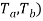2. Swap rounds(S,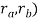3. Swap Teams(S,4. Partial Swap Rounds(S,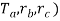5. Partial Swap Teams(S,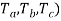After these Simulated algorithm is applied on the schedule which is obtained after implementation of above moves in order to obtain best feasible schedule. The cost objective function is used in order to calculate the best feasible schedules

Results of implementation of our Modified algorithm for TTP

 Linear Models Results BFC BFS BIC BIS NovBF NovBI 1.Constant Immigration and linear Emigration Model. 2. Linear Immigration and Linear Emigration Model. 3. Linear Immigration and Linear Emigration Model. 28757 29745 26701 <6*10 double> <6*10 double> <6*10 double> 2.1739e+04 2.0143e+04 2.0653e+04 <6*10 double> <6*10 double> <6*10 double> 0 0 0 16 16 16 Non Linear Models 4. Trapezoidal Migration Model 5. Quadratic Migration Model 6. Sinusoidal Migration Model 27765 27712 28708 <6*10 double> <6*10 double> <6*10 double> 2.0537e+04 2.1003e+04 <6*10 double> <6*10 double> <6*10 double> 0 0 0 18 18 18

Performance comparison of best feasible cost produced by linear and non linear-models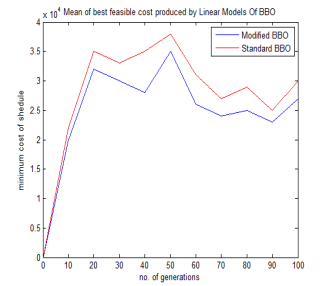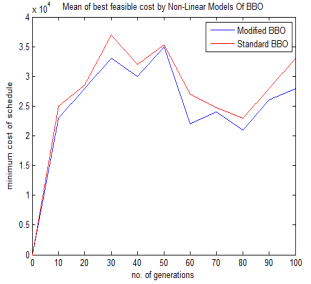## Related Services

View all

### DMCA / Removal Request

If you are the original writer of this essay and no longer wish to have your work published on the UKDiss.com website then please:

Related Services